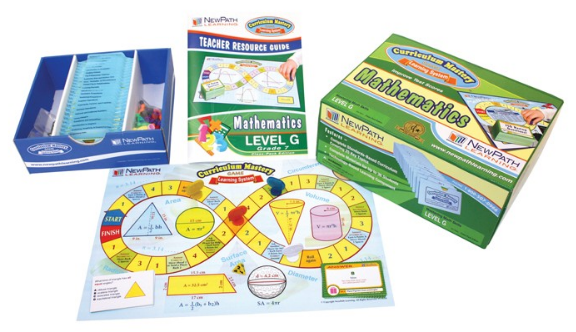# Tất cả sản phẩm# FLORIDA Grade 7 Math Curriculum Mastery® Game - Class-Pack Edition

Mã sản phẩm: W23-7061 Lược xem: 1318

Liên hệ

Includes 25 individually packaged sets of 30 colorful, review cards per topic - a total of 750 curriculum-based questions! Also includes 12 double-sided, laminated game boards; comprehensive Teacher Planning & Resource Guide; and FREE 1-Year Premium Subscription to NewPath's Online Learning Program providing access to Math games, interactive activities, quizzes and more. Complete curriculum coverage of the following Sunshine State Standards-based topics -

1. Organizing Data
2. Analyzing, Graphing & Displaying Data
3. Introduction to Algebra
4. Equations & Inequalities
5. Using Integers
6. Rational & Irrational Numbers
7. Decimal Operations
8. Exponents, Factors & Fractions
9. Fractions Operations
10. Numerical Proportions
11. Geometric Proportions
12. Introduction to Percent
13. Applying Percents
14. Plane Figures: Lines and Angles
15. Plane Figures: Closed Figure Relationships
16. Measurement, Perimeter & Circumference
17. Area & Surface Area
18. The Pythagorean Theorem
19. Finding Volume
20. Introduction to Probability
21. Using Probability
22. Algebraic Equations
23. Algebraic Inequalities
24. Introduction to Functions
25. Measurement Conversion